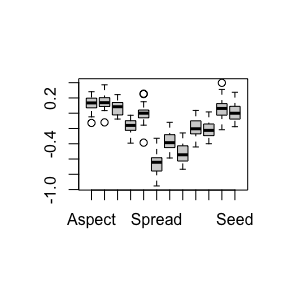# Aravo data analysis

library(lori)
set.seed(123)

# Brief data description

The Aravo data set (Choler, 2005) consists of three main data tables. First, a count table collecting the abundance of 82 species of alpine plants in 75 sites in France (the rows correspond to the environments, and the column to species). We will denote this abundance table $$Y\in\mathbb{N}^{n\times p}$$. Second, a matrix containing 6 geographical and meteorological characteristics of the sites. Third, a matrix containing 8 species traits (height, spread, etc.). We denote $$R$$ the matrix of row covariates, and $$C$$ the matrix of column covariates.

First, we put the data in the right shape to apply the lori function.

data(aravo)
Y <- aravo$spe R <- aravo$env
R <- R[, c(1,2,4,6)]
C <- aravo$traits d <- dim(Y) n <- d p <- d U <- covmat(n,p,R,C) U <- scale(U) # Multiple imputation with the lori method We start by tuning the parameters of the lori method # Tune regularization parameter res_cv <- cv.lori(Y, U, reff=F, ceff=F, trace.it=F, len=5) res_lori <- lori(Y, U, lambda1 = res_cv$lambda1, lambda2=res_cv$lambda2, reff=F, ceff=F) The multiple imputation function may be used to obtain intervals of variability for the estimated coefficients. The following command performs multiple imputation, with 20 replications. Then, one can visualize the variability of the main effects coefficients with a boxplot. # multiple imputation res_mi <- mi.lori(Y, U, lambda1 = res_cv$lambda1, lambda2=res_cv$lambda2, reff=F, ceff=F, M=20) #> 7% 13% 20% 27% 33% 40% #> Warning in stats::rbinom(n * p, 1, prob = (1 - probs)): production de NAs #> 46% #> Warning in stats::rbinom(n * p, 1, prob = (1 - probs)): production de NAs #> 53% #> Warning in stats::rbinom(n * p, 1, prob = (1 - probs)): production de NAs #> 60% #> Warning in stats::rbinom(n * p, 1, prob = (1 - probs)): production de NAs #> 66% #> Warning in stats::rbinom(n * p, 1, prob = (1 - probs)): production de NAs #> 67% 69% 70% 71% 73% 74% 75% 77% 78% 79% 81% 82% 83% 85% 86% 87% 89% 90% 91% 93% 94% 95% 97% 98% 99% boxplot(res_mi$mi.epsilon)